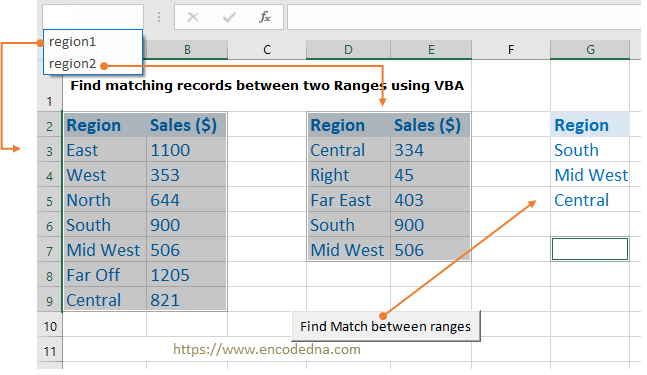﻿ Excel VBA to find Matching records between two Ranges

# Excel VBA to find Matching records between two Ranges

Let us assume, I have two ranges of values in my Excel worksheet. Each range has two columns each (region and sales). Now, both the ranges have some common regions. How do you find the matching records (the regions in particular) between both the ranges using VBA?I guess the image above explains what my macro will do. The ranges have three common regions. I want to extract the common regions out and show it in another column.

I am using a simple macro to do this and yes, the code has loops and its simple.

Note: I am assuming that you know what a Named Range is in Excel. If you don’t know what it is, please read this post first. You will understand.

The Macro
```Private Sub CommandButton1_Click()
Call findMatchingRecords
End Sub

Sub findMatchingRecords()
On Error GoTo ErrHandler

' Create range objects.
Dim rng1 As Range
Dim rng2 As Range>

' Assign both the defined ranges to the objects.
Set rng1 = Sheet1.Range("region1")
Set rng2 = Sheet1.Range("region2")

Dim iRow As Integer
iRow = 1

For Each rng1cell In rng1
For Each rng2cell In rng2
If rng1cell = rng2cell And rng1cell.Column = 1 Then             ' Will check only the first column (Region).
Sheet1.Cells(iRow, rng1cell.Column + 6) = rng1cell          ' Show the matching data.
iRow = iRow + 1
End If
Next rng2cell
Next rng1cell

ErrHandler:
Debug.Print Err.Description
End Sub```
The procedure findMatchingRecords() is called from inside a button click. You can call the procedure anyway, you like it.

First, I have created two Range objects and assigned ranges that I have created on my worksheet.

Next, I’ll iterate or loop through each cell in both the ranges using two For Each loop and compare the values. If it’s a match, I’ll write the values in another column (the 7th column).

That’s it.

Just remember, the records should fall inside the ranges. Make sure the named ranges are created properly.

If the two ranges are in two separate sheets, for example, region1 is in sheet1 and region2 is in sheet2, all you have to do is, assign the ranges accordingly inside the macro’s procedure.

```' Assign both the ranges.
Set rng1 = Sheet1.Range("region1")
Set rng2 = Sheet2.Range("region2")```

Thanks for reading.